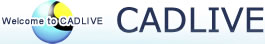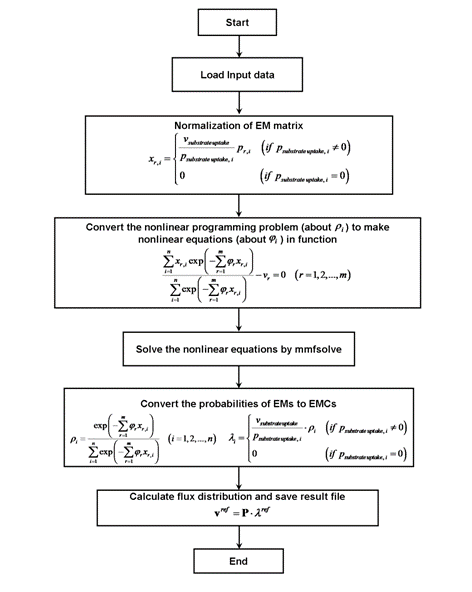### Use of maximum entropy principle extends the feasibility of elementary mode analysis

Elementary Mode (EM) analysis is potentially effective in integrating transcriptome or proteome data into metabolic network analyses and in exploring the mechanism of how phenotypic or metabolic flux distribution is changed with respect to environmental and genetic perturbations. The EM Coefficients (EMCs) indicate the quantitative contribution of their associated EMs and can be estimated by maximizing Shannon's entropy as a general objective function. We propose a fast and universal method that optimizes the EMCs under the constraint of the Maximum Entropy Principle (MEP). To demonstrate the feasibility of MEP, it is coupled with Enzyme Control Flux (ECF) to predict the flux distributions of E. coli and S. cerevisiae for different conditions (gene deletion, adaptive evolution, temperature and dilution rate) and to provide a quantitative understanding of how metabolic or physiological states are changed in response to these genetic or environmental perturbations at the elementary mode level.

References

Zhao and Hiroyuki Kurata, Use of maximum entropy principle with Lagrange multipliers extends the feasibility of elementary mode analysis", J. Biosci. Bioeng. 110: 254-261, 2010

The algorithms

(I) Large-scale optimization of Elementary Mode Coefficients (EMCs) by Maximum Entropy Principle with Lagrange Multiplier (MEPLM)
(II) Prediction of a flux distribution by Enzyme Control Flux (ECF).

Manuals of the programs

Program I: MEPLM_optimization.m

Input: MEPLM_optimization.mat
ems: whole matrix of elementary modes (reactions x elementary mode);
uptakeflux: flux for uptake reaction (1 x 1);
numuptake: the row in elementary mode matrix for uptake flux (1 x 1);
flux: flux for each reaction (1 x reactions);

Output: emc.mat
EMC: elementary mode coefficient (elementary mode x 1).

In matlab
>> MEPLM_optimization

ProgramII: ECFLM.m
Input: emm_flux_enzyme.mat, emc.mat
In emm_flux_enzyme.mat :
ems: whole matrix of elementary modes (reactions x elementary mode);
numuptake: the row in elementary mode matrix for uptake flux (1 x 1);
enzprofile: enzyme activity profile (reactions x 1);

In emc.mat:
EMC: the elementary mode coefficients optimized by MEPLM_optimization.m (elementary mode x 1).

Output: predictedflux.mat
predflux: predicted flux distribution (reactions x 1).

In matlab
>> ECFLMFigure Schematic diagram of the MEPLM program